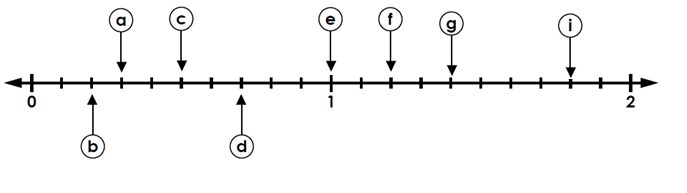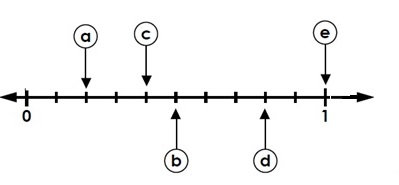# Reading Decimal Position on a Number Line: Tenths

Consider the number line given belowIt is divided into two major divisions 0-1 and 1-2. Each of this is further divided into 10 divisions which are tenths.

To read a decimal position of tenth on the number line consider some of the following examples. The letter a points to the third division between 0 and 1. So it represents 0.3. Similarly, b represents 0.2; c is 0.5 and d is 0.7.

e represents 1.0 or ten tenths and f, g and i represent values greater than 1.

f, g and i represent values 1.2, 1.4 and 1.8 respectively.

Identify the decimal numbers denoted by the letters on the number line given below.### Solution

Step 1:

a represents the second division so it shows the value two tenths or 0.2;

Step 2:

Similarly, b is 0.5; c is 0.4; d is 0.8 and e is 1.0

Identify the decimal numbers denoted by the letters on the number line shown below.### Solution

Step 1:

g, h, i and j represent values greater than 1.

Step 2:

g represents 1.1; h is 1.3; i is 1.6; j is 1.9